## Collocation BEM for 3D Lame Equation

Download CBEM_LAM, a package for solving the 3D Lamé equation based on a piecewise constant Collocation Boundary Element Method, and employing a boundary-only discretization technique.

# Piecewise constant collocation method

The displacementand tractionare assumed to be constant over each boundary element. It can be shown (see e.g. ) that the elastic potentials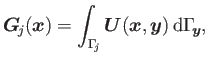and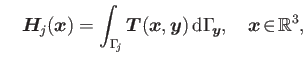due to a uniform distribution over a flat triangle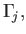can be employed to successfully computeandon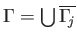. In addition, these potentials can be utilized to effectively calculate the displacementat interior points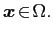The analytic expressions for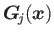and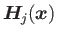over a flat triangle are given in . Moreover, due to its boundary representation, the elastic Newton potential can also be effectively evaluated without the need of a volume-fitted mesh . A brief description of the boundary-only discretization of the elastic Newton potential can be found in the user guide cbem_lamGuide.pdf provided in the package CBEM_LAM.

# References

 S. Nintcheu Fata.
Explicit expressions for three-dimensional boundary integrals in linear elasticity.
J. Comput. Appl. Math., 235(15):4480-4495, 2011.
 S. Nintcheu Fata.
Boundary integral approximation of volume potentials in three-dimensional linear elasticity.
J. Comput. Appl. Math., 242(1):275-284, 2013.